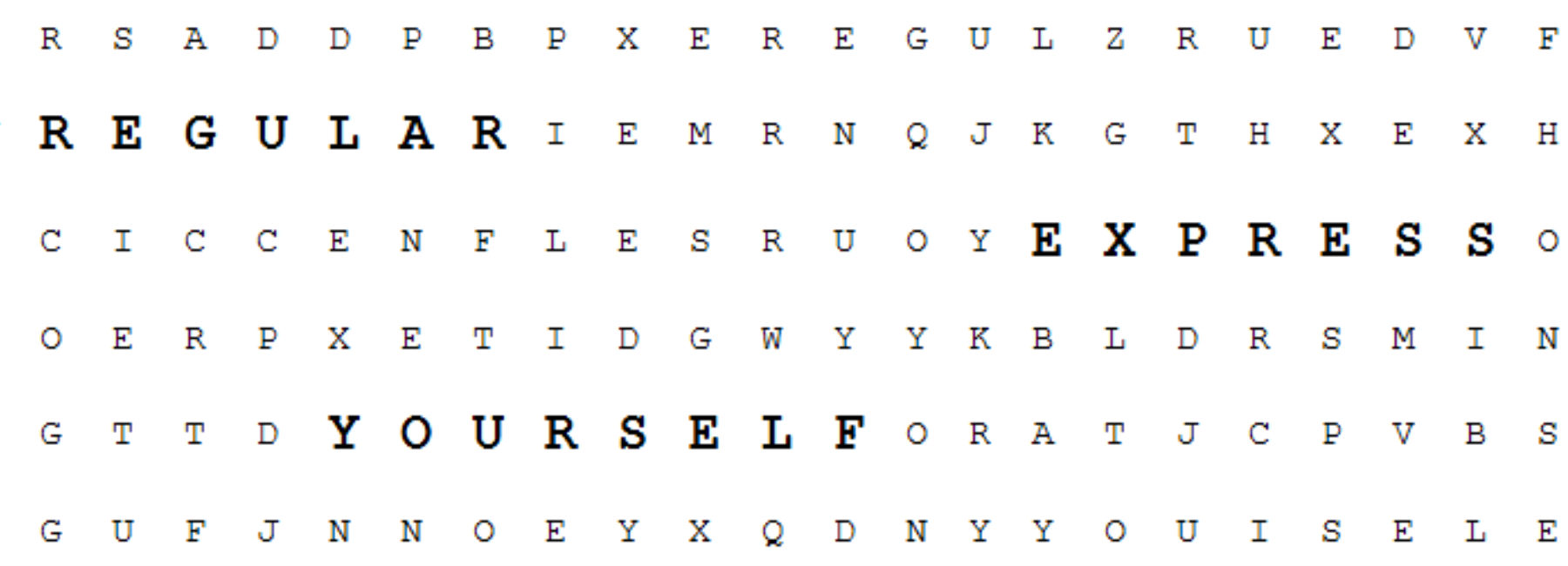# Regular Express Yourself using RegExpBuilder

Regular expressions.

There are typically two polarizing reactions to the above statement. The first may result in a charge of attempted murder against your mouse and the other may have nested closer to your screen saying "Yes? Tell me more!". This article is primarily going to focus on the first group of people that may currently be struggling to find their mouse from across the room.

Regular expressions can be incredibly powerful tools when dealing with large amounts of text and attempting to grab very specific data within it using different patterns or expressions. However, they are not the most friendly things in the world to look at or write :

``````// Example of an incredibly ugly regular expression
// to match dates in a variety of formats
((0?|10|12)(-|/)((0[0-9])|()([0-9]?)|(3?))(-|/)((d{4})|(d{2}))|(0?|11)(-|/)((0[0-9])|()([0-9]?)|(3?))(-|/)((d{4}|d{2})))
``````

This post is going to cover a new library called RegExpBuilder that was released by Andrew Jones, which aims to transform these very nasty looking regular expressions into human friendly statements that can easily be built and understood.

#### The Problem

You need to write a very basic regular expression to perform some pattern matching and you don’t have any idea how to write a regular expression (or you do and they always turn out wrong).

#### Using RegExpBuilder

RegExpBuilder can target a variety of environments such as Dart, Java, Javascript and Python. For this post, we will focus on the use of Javascript since it will be very easy to demonstrate through the use of examples that would be at least somewhat interactive.

Getting started with RegExpBuilder is as simple as including the appropriate file or reference into your application (based on your environment) like so :

``````<!-- Example of directly referencing the RegExpBuilder.js file from github -->
<script type='text/javascript' src='https://raw.github.com/thebinarysearchtree/RegExpBuilder/master/RegExpBuilder.js' />
``````

Let's look at a few examples that will compare and contrast a few common regular expressions with those constructed using RegExpBuilder to get an idea of how things look. You can check out the available documentation here as well, which might be helpful when reviewing over these basic examples.

#### Dealing with Currency

A common regular expression might be to validate if a value contains is currency or not. In this example, we will consider currency to be US dollars which will consist of an explicit dollar sign '\$' followed by a series of numbers, then a dot '.' and exactly two decimal places such as:

``````\$123.45 # Perfect example of a US currency value
``````

Using a Regular Expression, you would get something that looks like this :

``````^\$\d+.\d{2}\$
``````

Let's break this down for those of you unfamiliar with regular expressions :

``````^      # Start of Expression
\$      # An explicit '\$' symbol (escaped with a slash)
\d+    # One or more digits (digits denoted by the d and one or more indicated by the '+')
.      # An explicit '.' symbol (this must be escaped as '.' matches a variety of characters in Regular Expressions)
\d{2}  # Exactly 2 digits (notice the digit symbol from earlier followed by the braces used to denote quantity)
\$      # End of the expression
``````

and the same thing would look like this when built through RegExpBuilder :

``````// Constant collection of digits (this will be used
// throughout these examples)
var digits = ["0", "1", "2", "3", "4", "5", "6", "7", "8", "9"];

var regex = new RegExpBuilder()
.startOfLine()           // ^
.then("\$");              // \$
.some().from(digits)     // \d+
.then(".")               // .
.exactly(2).from(digits) // \d{2}
.endOfLine()             // \$
.getRegExp();            // (builds the regex)
``````

You should immediately notice the difference in readability. The beauty of RegExpBuilder is that it actually reads extremely well, which translates into it being easily written. Now let's use a very simple Javascript alert to see what the RegExpBuilder generates for us through a simple `alert(regex)` call: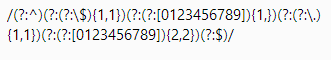Basically, they operate in the exact same manner as traditional Regular Expressions, but they simply maintain a higher level of readability when being generated.

#### Dealing with Basic Phone Numbers

Phone numbers are another common use-case when discussing regular expression-based validation. Although they can be terribly complicated, we will define a very basic one for demonstration purposes :

``````555-555-5555 # A very common US Phone number example
``````

A the expression for which might look like this :

``````^\d{3}-\d{3}-\d{4}
``````

and could be explained via the following breakdown :

``````^      # Start of Expression
\d{3}  # Exactly three digits (area code)
-      # An explicit '-'
\d{3}  # Exactly three more digits (first component of phone number)
-      # Another hyphen
\d{4}  # Exactly four digits
\$      # End of the expression
``````

Not too tough right? Let’s try it with RegExpBuilder…

``````var dashes = new RegExpBuilder()
.exactly(3).from(digits).then("-")  // \d{3}-
.exactly(3).from(digits).then("-")  // \d{3}-
.exactly(4).from(digits)            // \d{4}
.getRegExp();
``````

which would render the following if we used another `alert(regex);` call :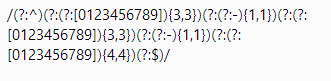That isn't very interesting though is it? How about a slight change to allow for optional area codes like these :

``````555-5555     # Valid
555-555-5555 # Valid
``````

which would have an expression that looks like :

``````^(\d{3}-)?\d{3}-\d{4}\$
``````

The only changes that are being made from the previous example is that we are grouping our first section using parentheses and indicating that this group can only appear 0 or 1 times (i.e. optional) :

``````(\d{3}-)?
``````

You'll find that the RegExpBuilder allows you to create other RegExpBuilder objects that can be passed in as groups to allow you to easily separate all of the components when dealing with complex expressions through the `like()` function :

``````// Build our first section (the optional area code part)
var areacode = new RegExpBuilder()
.exactly(3).from(digits).then("-");  // \d{3}-

// Build a Regular Expression to validate against using
// the RegExpBuilder
var regex = new RegExpBuilder()
.startOfLine()                          // ^
.min(0).max(1).like(areacode).asGroup() // (\d{3}-)?
.exactly(3).from(digits).then("-")      // \d{3}-
.exactly(4).from(digits)                // \d{4}
.endOfLine()                            // \$
.getRegExp();
``````

which functions identically to the existing Regular Expression above and generates the following :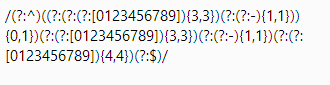#### Dealing with Advanced Phone Numbers

How about adding even more flexibility to it so that it could accept periods '.' or spaces ' ' between the values and an optional area code like the following examples :

``````555.555.5555 # Acceptable
555-5555     # Acceptable
555 5555     # Acceptable
5-5-5-5-5-5- # Obviously not acceptable
555.555-5555 # Judges? Nope. Not allowed.
``````

An important factor to remember here is that we want consistency and don’t want different symbols being mismatched like in the last example above, so we will separate the expression into three parts (one to handle dashes, another to handle white-space and another to handle periods) and we should end up with something like this :

``````^(((\d{3}-)?\d{3}-\d{4})|((\d{3}\s)?\d{3}\s\d{4})|((\d{3}.)?\d{3}.\d{4}))\$
``````

Rather than typing a page-long character-by-character breakdown, I’ll summarize it as follows :

``````^                        # Start of Expression
(                        # Wraps all of the expressions
((\d{3}-)?\d{3}-\d{4})   # Takes care of dashes-format
# with optional area code (notice
# the ? behind the first "group")
|                        # An explicit OR
((\d{3}\s)?\d{3}\s\d{4}) # The white-space group (s denotes
# white space)
|                        # Another OR
((\d{3}.)?\d{3}.\d{4})   # The period notation (. is an
# explicitly escaped dot)
)                        # Closes the outer "wrapper"
\$                        # End of expression
``````

Now we are going to get into some real complexity, but at least it will be somewhat human readable :

``````// Handle prefixes (optional area codes for each format)
var areacode_dash = new RegExpBuilder().exactly(3).from(digits).then("-");  // \d{3}-
var areacode_space = new RegExpBuilder().exactly(3).from(digits).then(" "); // \d{3}\s
var areacode_dot = new RegExpBuilder().exactly(3).from(digits).then(".");   // \d{3}.

// Build each of the individual components (dashes, spaces and dots)
var dashes = new RegExpBuilder()
.min(0).max(1).like(areacode_dash).asGroup()  // (\d{3}-)?
.exactly(3).from(digits).then("-")            // \d{3}-
.exactly(4).from(digits);                     // \d{4}

var spaces = new RegExpBuilder()
.min(0).max(1).like(areacode_space).asGroup()  // (\d{3}\s)?
.exactly(3).from(digits).then(" ")             // \d{3}\s
.exactly(4).from(digits);                      // \d{4}

var dots = new RegExpBuilder()
.min(0).max(1).like(areacode_dot).asGroup()  // (\d{3}.)?
.exactly(3).from(digits).then(".")           // \d{3}.
.exactly(4).from(digits);                    // \d{4}

// Handle build final expression
var regex = new RegExpBuilder()
.startOfLine()             // ^
.eitherLike(dashes)        // ((\d{3}-)?\d{3}-\d{4})
.orLike(spaces).asGroup()  // |((\d{3}\s)?\d{3}\s\d{4})
.orLike(dots).asGroup()    // |((\d{3}.)?\d{3}.\d{4}))
.endOfLine()               // \$
.getRegExp();
``````

and testing it out, let's see what it yields :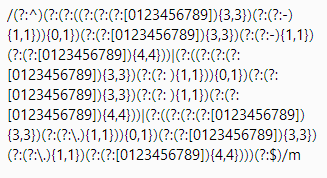Holy moly. Although that may be an incredibly large expression, it actually works just as the plain expression presented earlier and reads well in English.

#### Summary and Code Examples

think one of the most important things to take away from this library is that it isn't for everyone. If you know you way around working with regular expressions, it'll likely take up more of your time than necessary. This is geared towards those that aren't fond of working with traditional regular expressions and want to have a method for writing and using them in a very generic and human-readable way.

It would be a great tool to use for improving maintainability within large scale projects that relied heavily on the use of expressions so that developers wouldn't have to go "what the hell does this gibberish do?". Obviously, due to the nature of the beast, these expressions aren't optimized by any means as this library clearly focuses on improving readability over performance. I'm sure there are plenty of folks out there that would love to expand upon something like this and possibly extend it to be more optimized, flexible or whatever your heart desires.

If you enjoyed this post or it sparked an interest in you, feel free to check out the project on GitHub. I’ve also created an example project that contains all of the examples that are found within this post as well to allow you to tinker with as you please :